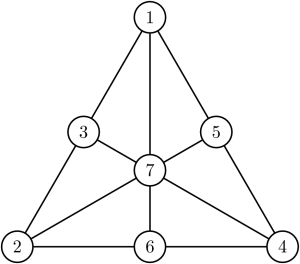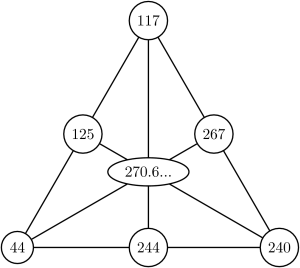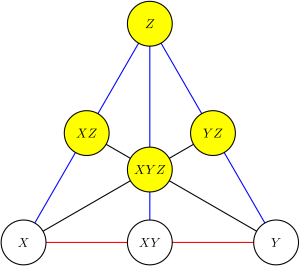# Jos’ Perfect Cuboid

Inspired by our Open Season post on the Perfect Cuboid earlier this year, Aperiodical reader Jos Schouten wrote to us describing his work on the problem over the past 20 years. He’s looking for someone to help take his work further. Are you up to the challenge?

## Survey of the Perfect Cuboid

This article is about my search for the Perfect Cuboid (PC), which started exactly on Wednesday April 15, 1987. At that time I was a young engineer with feelings for mathematics, and employed to write C-language programs on a UNIX platform. Since then I’ve written software and explored ideas to find the cuboid, at work and at home. I still haven’t found one!

This article is also hoping to find someone in the world community as sparring partner, who likes the subject, wants to propose additional solution methods, and can help to implement such a method. The attempt will be to find a perfect cuboid with an odd side less than a googol.

### Statement of the Problem

The problem is easy to state, extremely difficult to solve, but a solution, once found, would be easy to verify.

The problem in words:

Find a cuboid whose sides are integer lengths, whose face diagonals are integers and whose space diagonal (from corner to opposite corner) is integral too.

The problem can be phrased in a picture as follows: put numbers in the circles so that for each straight line, the squares of the two numbers at the ends of the line add up to the square of the middle number.To help understand this, I first give an example of the problem where addition-with-squaring is replaced with normal addition.

Seven numbers must be entered in the circles. On each of the six straight lines you find three circles. The number in the middle circle on a straight line must be the sum of the other numbers on the same straight line. There are infinitely many solutions to this problem, but the one shown in the picture is the most simple, smallest and most elegant.

The Perfect Cuboid problem can be visualised in the same way.Seven numbers must be entered in the circles. On each of the six straight lines you find three circles. The number in the middle circle on a straight line must be the squared sum of the other numbers on the same straight line.

Example: $44^2 + 117^2 = 125^2$. This solution was found by Euler. The circles with the numbers $44$, $117$ and $240$ represent the sides. The circles with the numbers $125$, $244$ and $267$ represent the face diagonals. The middle number is the space diagonal. The solution found by Euler has integral sides and face diagonals, but the space diagonal is not integral.

To solve the Perfect Cuboid problem, all seven numbers must be integral.

### Odd and Even Sides

If the lengths of the sides of the cuboid have no common factors, this is called a primitive perfect cuboid. In this case, one side must be odd and the others are even.

If someone found a perfect cuboid, and told me the size of the odd side, then I could calculate the other sides, as follows.The sides of the cuboid are named $X$, $Y$ and $Z$. The face diagonals are named $XY$, $XZ$ and $YZ$. The space diagonal is named $XYZ$. Side $Z$ is arbitrarily chosen to be odd, and is coloured yellow. Then $XZ$, $YZ$ and $XYZ$ are odd too, and the others are even.

Now look at the three blue lines, representing the equations

\begin{align}
Z^2 + X^2 &= XZ^2 \\
Z^2 + Y^2 &= YZ^2 \\
Z^2 + XY^2 &= XYZ^2
\end{align}

In general, $Z^2 + E^2 = O^2$, where $E$ is an even number and $O$ an odd number. In other words: for an odd number $Z$ there must be at least three even numbers ($X$, $Y$ and $XY$) fulfilling the above equations. Suppose for the given $Z$ one million even sides $E$ can be calculated. The only thing I have to do is select three of them such that the red equation is also fulfilled, i.e. $E_i^2 + E_j^2 = E_k^2$. Then $X = E_i$ and $Y = E_j$.

The above was my approach in 1987, and it still is.

The process breaks down into four stages:

• select $Z$
start with $Z=3$. Then select $5, 7, 9, 11, \ldots$
• calculate all $E$
$E = \frac{\frac{Z^2}{d}-d}{2}$, where $d$ is a proper divisor of $Z^2$, less than $Z$. So all proper divisors $d$ need to be generated. This can be done fast if the prime factors of $Z$ are known, so I start by factorizing $Z$.
• sort $E$
The disadvantage of the fast $E$ calculation is that the list of $E$ values is not in ascending order – at least, I don’t know a way to quickly generate a sorted $E$ list. The sorting is necessary to make the next step possible within a feasible timescale.
• red check
The last step is to verify if $E_i^2 + E_j^2 = E_k^2$. If so, we have found a perfect cuboid.

Note that in 1987 GMP – the GNU Multi precision arithmetic library – was not invented yet. So I defined my own big unsigned integer of 128 bits, and wrote functions to add, subtract, multiply and print them. It worked, and I have tested $Z$ up to approximately $10^9$ – of course, without success.

### Current Work

I have bought a 2TB external hard disk to store $E$ values, using the compact raw format of GMP. I have selected a $Z$ with as many prime factors as possible. This is:

$Z = 3^2 \cdot 5^2 \cdot 7 \cdot 11 \cdot 13^2 \cdot 17 \cdot 19 \cdot 23 \cdot 29 \cdot 31 \cdot 37 \cdot 41 \cdot 43 \cdot 47 \cdot 53 \cdot 59 \cdot 61 \cdot 67 \cdot 71 \cdot 73 \cdot 79 \cdot 83 = 26038790279704395507173341754297025.$

This gives 72,641,341,687 $E$ values, stored in 7,265 files, each 10 million numbers. The sorting is problematic under Microsoft Windows. The process involves a lot of copying files between my hard disk and the external hard disk. But those copies are not reliable under Windows: there are bit errors without any warning. Now I have ported the whole to a Linux platform. Still busy.

What would be very helpful is if someone knows a fast way to generate a sorted list of values of $E$.

Do you think you can help Jos? If so, please write a comment below or contact root@aperiodical.com.

•### 24 Responses to “Jos’ Perfect Cuboid”

1.Matt E

I’m more of a mathematician than a computer scientist, so at this point my efforts would go toward trying to prove that no such PC exists.

•Jos Schouten

If you can prove that $[\frac{ab}{a^2-b^2}]^2+[\frac{cd}{c^2-d^2}]^2=[\frac{ef}{e^2-f^2}]^2$ has no solution for integral $a$, $b$, $c$, $d$, $e$ and $f$ than you have proven that no PC exists!

•satyam

io have proved that no a perfect cuboid doesnt exist but i dont whom to submit my research paper

2.Paul Coombes

A very minor point but shouldn’t the bottom, left corner of the last diagram be labelled with an X not a Z?

•Christian Perfect

Whoops! That’s my fault. Fixed now. Thanks for pointing it out.

3.Andrew

An even more minor point, but there’s an extra factor of 13 in the value given for Z.

4.Thomas Egense

A question about the sorting of the E-values.

If you had the proper divisors (d) in Z*Z sorted, wouldn’t that also produce the
E-values in sorted order?

•Anonymous

Yes, if the proper divisors are sorted the E-values are sorted as well.

•Jos Schouten

Yes it will.

5.Tim Roberts

I’m a little concerned when you say you’ve tested up to 10^9. The odd side has already been searched up to 3*10^12, with no solutions found. See
http://www.durangobill.com/IntegerBrick.html
This and other problems are on the Unsolved Problems web site at
http://unsolvedproblems.org/
and are discussed on the associated Yahoo! group at
http://tech.groups.yahoo.com/group/UnsolvedProblems/

You’d be very welcome to join the group and discuss your ideas!

Tim

•Jos Schouten

Well, I tested up to 10^9 in 1987-1988. At that time I was not aware of the attempts of Durango Bill.
I had a look on the Yahoo! group (last link), but there is not much to see. Should I first join the group before I get some info?

6.Thomas Egense

Here is another useful page with information about “3D perfect Euler Brick” and also list the 3*10^12 brute force attack.
http://www.christianboyer.com/eulerbricks/index.htm#3D

The website also list many other interesting unsolved problems involving square numbers.

7.Nick Birks

I think I found a perfect cuboid and I have questions. What is the prize for the answer and is there someone I should send the answer to someone?

•Christian Perfect

It should be very easy to check if you have found one. I’m not aware of any serious prize being offered for finding a perfect cuboid. The owner of unsolvedproblems.org claims to be offering \$500 prizes for each of the problems on the site, of which finding a perfect cuboid is one, but I’m not sure how serious the offer is. Reply •Tim Roberts Very serious! And in fact I’ve raised the prize for either finding a perfect cuboid, or alternatively proving that a perfect cuboid cannot exist, to$1000.

•A.Sarafov

I want to contact Jos Schouten the author.If you want get in touch with me so we can talk about PC I have a proof! Thank you in advance and one more thing \$1000 for the task is peanut.

•Mark Tarrabain

There are, in my view, two problems with the organization of unsolved problems for awarding those types of prizes for these problems.

The first and probably more cynical-minded issue is that the amount they are offering is not an amount that a person who who might be qualified to design solutions would find worthwhile to justify the expenditure of any effort unless they already happen to have a solution. This means that submissions to the site are liable to largely be by amateurs, and in turn this leads to the second problem.

The second issue is that in order to even claim the prize at all, one’s solution must be accepted by the mathematics community within 18 months of its first publication, which evidently also means within a year and a half of being published on that site. If a person has so few connections in the mathematics community that they would resort to using such a site to first publish their work, it is quite likely they may not have been able to get their submission reviewed by the community in the first place, so an otherwise potentially legitimate proof may go completely unobserved and unrewarded.

8.Ark-kun

>”The sorting is problematic under Microsoft Windows. The process involves a lot of copying files between my hard disk and the external hard disk. But those copies are not reliable under Windows: there are bit errors without any warning.”
Sorry, but that cannot be true. People (me included) included are moving many terabytes a day and never have a single corruption (checked using MD5 hashes).
You probably have hardware problems like faulty RAM.

9.richard

Very interesting, I’m not exactly the CS type, I’m actually attempting to find a proof that no perfect cuboids exist.

My attempt at proof uses a well known property of perfect squares:

1+3+5+…+No = N^2

Where No is the Nth odd in a consecutive sum.

Basically if you can find 6 perfect squares, one nested within another in a consecutive sum like this:

1+3+5+…+[N1+…+[N2+…+[N3+…+[N4+…+[N5+…+[N6+…+Ro]]]]]]

You would find a solution to the set of equations that represents a perfect cuboid.

If you are interested in a more thorough derivation I’d be happy to provide. Its good to see some people interested in this problem.

10.Rob Matson

Just an update on what Tim Roberts posted in October of 2013. I’ve been working the perfect cuboid problem (via computer) for about 3 1/2 years. Extending on Bill Butler’s work, I have exhaustively tested all odd sides up to 8.6 trillion (8.6E+12) and found no perfect cuboids. (I will continue this search at least until I reached 10 trillion). More recently I’ve been extending the minimum possible even side for a perfect cuboid. This is a slightly slower search, so it will take many years to reach the current minimum odd side. The prior published value for the minimum side was 10^10 (10 billion) which was recently significantly improved upon by Randall Rathbun to be at least 70 billion. My current search has more than tripled this minimum to 220 billion.

11.Michael Ejercito

Proving the opposite- that the perfect cuboid can not exist would likely require a proof by contradiction. Either we assume one exists, and conclude something that contradicts the original premise (like the smallest primitive perfect cuboid has an oblique angle, or that an even smaller primitive perfect cuboid can be implied) or that it contradicts an established fact (e.g., assuming the existence of a perfect cuboid would lead us to conclude that pi is rational, or that a counterexample to Fermat’s Last Thereom exists.)

(Note that the irrationality of pi and Fermat’s Last Theorem were both proved with contradictions.)

12.Number's lover